Home MathLab Solving Polynomial Equations Using Matlab

# Solving Polynomial Equations Using Matlab

Polynomial equations are some of the most popular types of equations in Math. Knowing how to solve them is a thing but actually solving them is another thing.

The methods you can use to solve them are many, but if you happen to have Matlab or the free Matlab alternative Octave you might as well be good using them to buy time if the purpose of solving the equation is more than simply solving the equation.

Caution: The following methods will help you solve polynomial equations quickly but will not show you how to manually solve polynomial equations, it will simply help you get results faster. This can be helpful if you are not interested in the method but on the answers and may also be very useful to check your result after manually solving your equations.

## Solving polynomial equations using Matlab

### Solving quadratic equations using Matlab

Quadratic equation are equations in the form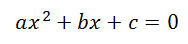Where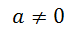So you will also find quadratic equations in the form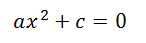or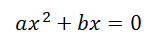Let’s go ahead and solve the following equation with Matlab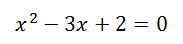To solve this equation with Matlab you will enter the following code

`roots([1 -3 2])`

and Matlab will give you the roots of the polynomial equation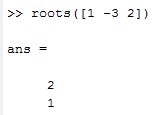If the equation was the following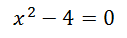the code would be

`roots([1 0 -4])`

and the result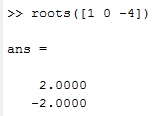### Solving cubic equations using Matlab

Let’s use the following equation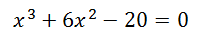The line of code to solve it won’t be that different compared to the previous one. The only difference here is that we have non-zero third-order coefficient to add to it

`roots([1 6 0 -20])`

Do not forget to add 0 between 6 and -20 since the first-order coefficient is zero

The result will be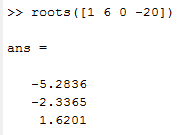### Solving quartic equations using Matlab

Using the following polynomial equation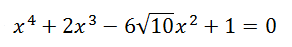The code will be

`roots([1 2 -6*sqrt(10) +1])`

And the result will be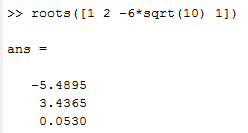The higher-order the higher number of coefficients. Remember the order which with you enter coefficients in the code affect the result, and always remember to put 0 to indicate where the coefficient is not existent for lower exponents of the equation.